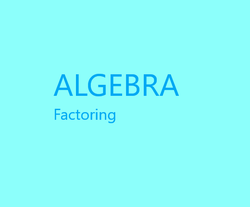# The Retired Professor Simplifies Algebra: Factoring

## This algebra insight is intended to assist students and those helping students with the learning process. It is a free to use resource. This snipet covers factoring.

Factoring can be thought of as undoing polynomial multiplication. It is therefore important that polynomial multiplication, presented in an earlier work, be understood prior to undertaking a study of factoring.

Factoring then becomes a tool for solving higher order equations by reducing them to easier to solve equations, often linear equations.

Factoring also allows us to utilize a concept called completion of the square. This technique allows us to develop the extremely important quadratic formula, and to work with the conic sections of the circle, the ellipse, the parabola, and the hyperbola. So, factoring should not be taken lightly.

### Factoring Techniques

First, we answer the question, “What is factoring used for?”

Students need to be given a reason for studying.  The answer here is this is a powerful tool that can be used in equation solving.

In factoring we break down expressions to two or more expressions that are multiplied by each other.  The parts used in that multiplication are called factors.

First, remove any common factor.

Factor 5x3 + 5x2 + 25x.  each term contains 5x, so it can be removed as a common factor.

5x(x2 + x + 5)

It is important that common factors be removed first, since doing this makes the problem easier.

Factoring by grouping is a form of removing common factors.

Factor x3x2 + 7x – 7

Group the first two terms, and also group the second two terms.  Take out any common factor from the group.

x2(x – 1) + 7(x – 1)

Notice x – 1 is a common factor.

(x – 1)(x2 + 7)

It should be pointed out that two groupings will work, but the third does not.  So, if pairing terms one way does not work it may be that pairing terms in a different way will be worth doing.  In an advanced approach terms can be grouped three or more at a time into a single group.

Factoring a trinomial is not always possible, but the technique to factor trinomials is well worth learning.

Factor x2 - 3x – 4

Note that the negative signs are parts of the numbers.  Algebra is built on two operations, addition and multiplication, and subtraction is thought of as adding a negative number.  Likewise, division is thought of as multiplying by a reciprocal.

We want two numbers that add to -3, and multiply as -4.

(x + a)(x + b) = x2 + ax + bx + ab = x2 + (a + b)x + ab

So, we need two numbers that multiply together to give, in this case, -4 and 1.  The possible number pairs are (-1, 4), (-2, 2), and (-4, 1).  In order to get the middle term to be -3x, we need that pair of numbers from the list that add to -3.  That is -4 and 1.

x2 - 3x – 4 factors as (x – 4)(x + 1).

When x2 has a coefficient it also must also be factored.  However, changing all of the signs gives nothing new, so it need not be done for the lead term.

Factor 2x2 – 5x + 3.

The possible pair for the 2 is (2, 1).  (Notice -2, -1 will lead to repetitions.)  The possible pairs for the 3 are (-1, -3) and (1, 3).  Now we must be careful where we place things.  The -5 must come from multiplying factors of the 2 by factors of the 3.  2(-1) and 1(-3) add together to get

-2 + (-3) = -5.

Remember, we are undoing polynomial multiplication.

(2x - 3)(x - 1) has the 2(-1) and the 1(-3).  Proper placement is critical.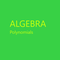This of a free resource where insights into algebra are provided to help both students and those helping students with algebra.

### Special Factoring

The below are well worth knowing.  They come in handy.

x2a2 = (xa)(x + aDifference of two squares

x2 + 2bx + b2 = (x + b)and x2 - 2bx + b2 = (x - b)are perfect square trinomials.

The above special cases can be factored using trinomial factoring.  But we often use them is other settings.

x3 + a3 = (x + a)(x2ax + b2) and x3 - a3 = (x - a)(x2 + ax + b2) are the factoring for the sum and for the difference of cubes, respectively.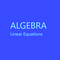Algebra simplified into a brief but thorough free resource can benefit both a parent helping a student and the student. This segment addresses linear equations.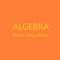This is the second part of a free resource to help with the basic understqanding of algebra. It can be used by students and those helping students witht the understanding.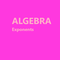This is a free resource on understanding algebra, and presented in a short presentation aimed at making things clear. It may help thoses studying algebra and those trying to help.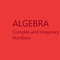This is a free to use algebra resource. It is designed to make it easy to understand complex and imaginary numbers for students and those assisting students with learning.

This article contains links to affiliate programs and Adsense advertising.  These must use cookies to allow for proper crediting, and allow me to earn from qualifying purchases. As an Amazon Associate I earn from qualifying purchases.

Updated: 02/09/2021, blackspanielgallery
1

Only logged-in users are allowed to comment. Login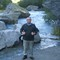frankbeswick on 02/11/2021

Educators should commit themselves to life long learning, but not all do. I have encountered one or two whom I would call ignorant.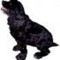blackspanielgallery on 02/10/2021

Unfortunately, in this state it is more so than in most. Tense id not important to many of them. And care for the math is also often lacking. Our universities have a wide range from academic excellence to what I would call diploma mills. I taught where we hired some weak people from one certain university, and it was obvious.frankbeswick on 02/10/2021

I was for a short time copy editor of a school newspaper and my experience confirms that not every teacher has writing skills.blackspanielgallery on 02/09/2021

Each state handles education differently, and some introduce advanced topics earlier than others. I once reviewed textbooks intended for use in various states, and all is not equal. In fact, the teachers who contributed to those books had different skill levels, some had difficulty keeping a paragraph in present, past, or future tense, and jumped from one to another. Others had teachers who could actually write English.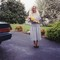DerdriuMarriner on 02/09/2021

blackspanielgallery, Thank you for the practical information and the product line.
How early is factoring being introduced in schools? Master gardeners and master naturalists here may fulfill their yearly Cooperative Extension hours through helping to teach fourth-grade science. Fourth-grade science seems like middle school although the students handle the leap well since the commonwealth school system prioritizes quality teachees and teachers.

#### You might also like

Learn Algebra and Trigonometry with Rap Videos

Learn the hard to grasp Algebra and Trigonometry basics from inspiring math t...

The Retired Professor Simplifies Algebra: Systems of Equations

Insights into algebra with attention to systems of linear equations is the fo...

The Retired Professor Simplifies Algebra: Exponents

This is a free resource on understanding algebra, and presented in a short pr...

Disclosure: This page generates income for authors based on affiliate relationships with our partners, including Amazon, Google and others.